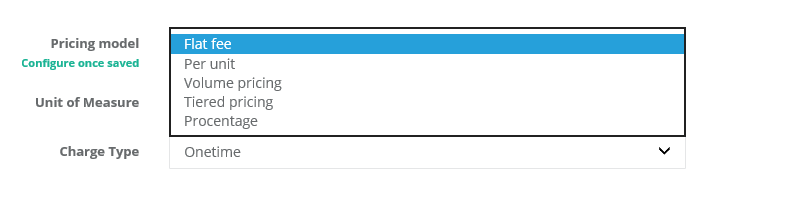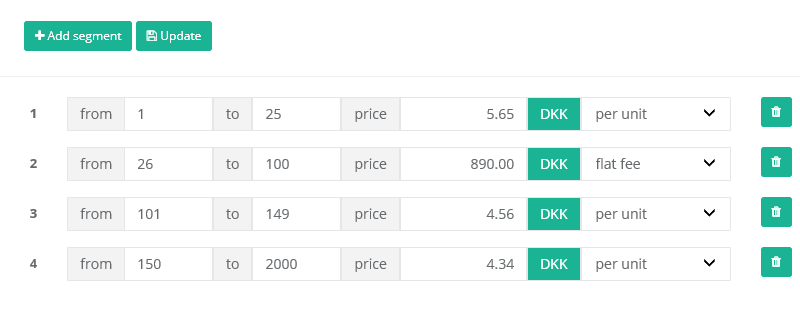# Pricing models

Upodi provides support for various monetization models. A monetization model is componentized by a pricing model and a charge type.

Upodi support

The following outlines the different use.Due to support of underlying finance systems (ERP), Upodi will handle all decimals when calculating tier and volume pricing, by supplementing quantity with 1 x the calculated price and adding the full quantity of this to the invoice line. ERP systems often calculate pricing of amount x quantity price, rendering the full amount wrong if decimals becomes larger than 2.

### Flat-fee

A flat fee pricing model bills a subscription a fixed amount in the term of the subscription. Flat fee pricing models ignore the quantity of the subscription charge, however, a subscription charge quantity of 0 will ignore the fee in a billing cycle.

Flat fee pricing models are usually used for sign-up fees, cancellation fees and based level subscription fees.

### Per-unit

A per-unit pricing model, multiplies the per-unit amount with the quantity of a subscription charge. The following formular is used

``````quantity * per-unit price
``````

Per unit fees are often used in SaaS subscription plans (per user) or mobile data plans (per gb data).

### Tiered pricing

A tiered pricing model offers a flexible billing based on the quantity amount of a subscription. A tiered pricing model is calculated based on the quantity tier and type of that tier multiplying each tier of the full quantity.Each tier can be charged by per-unit pricing level (see above), or a Flat fee level (see above).

#### Example:

A tiered pricing model is calculated by the sum of all tiers matching the volume. Let's try to define this by looking at a sample table.

Tier

Pricing level

Pricing

Range

A

Per-unit

\$20 per unit

1-100

B

Per-unit

\$15 per unit

101-200

C

Per-unit

\$10 per unit

201-300

A tiered pricing model for a quantity of 130, will sample calculation of tier A plus tier B but not tier C. Calculation is done as follows:

``````Tier A = 100 * \$20 (per unit) = \$2.000
+
Tier B = 30 (remainder) * \$15 = \$450
================
Totals = \$2.450
``````

### Volume pricing

A volume pricing is often called a "discount ladder". A volume pricing models looks for a given tier of the volume pricing model, and calculates the total quantity of this tier.

#### Example

Using the sample table from above (tiered pricing), a volume pricing model, calculated with a quantity of 130, is done as follows. 130 matches tier B:

``````Tier B = 130 * \$15 = \$1.950
================
Totals = \$1.950
``````

### Procentage total

Upodi only support one Procentage total pricing model per product plan. In addition, the Procentage total pricing model, is the last pricing model to be calculated on if present.

The calculation of this pricing model, is done as a procentage of total, of all previous charges summed together. Hence the name.

``````Sum of all previous charges * %procentage pricing model
``````

The Procentage total pricing model, can be used to apply credit card (i.e. 0.5%) feeds or typed discounts onto a product plan.

## Questions?

We're always happy to help with code or other questions you might have! Search our documentation or contact helpdesk. You can also chat live with us using the Intercom icon.